All submissions of the EM system will be redirected to Online Manuscript Submission System. Authors are requested to submit articles directly to Online Manuscript Submission System of respective journal.

# A Flux Formula For Weighted Manifolds

Abdelmalek M*

High School of Management, Aboubekr Belkaid University, Tlemcen, Algeria

*Corresponding Author:
Abdelmalek M
High School of Management, Aboubekr Belkaid University, Tlemcen, Algeria
E-mail: [email protected]

Received Date: 02/03/2017; Accepted Date: 17/03/2017; Published Date: 22/03/2017

Visit for more related articles at Research & Reviews: Journal of Statistics and Mathematical Sciences

## Abstract

In this article, we derive a flux formula in a weighted manifold, using the weighted Newton transformations and introducing the notion of weighted higher order mean curvature, this formula generalizes. Reilly’s original formula and the flux formula obtained by Alias, Lopez and Malcarne . In particular, we obtain a similar balancing formula obtained by Rosenberg. Finally, we give some special cases of our formula.

#### Keywords

Weighted manifolds, Weighted, Newton transformation, rth mean curvature, Weighted mean curvature

#### Introduction

Most of the useful integral formulas in Riemannian geometry are obtain by computing the divergence of certain vector fields and then apply the divergence theorem. In the authors gave a flux formula. In particular, they derive a similar balancing formula given by Rosenberg . As an application to this flux formula they gave an estimation of the higher order mean curvature Hr of a hypersurface in space forms by the geometry of its boundary . Motivated by the work of these authors, we give in this work a flux formula in the case of weighted manifolds. Recall that a weighted manifold (called also a manifold with density) is a Riemannian manifold M endowed with a smooth positive density e-f with respect to the Riemannian measure. We proof the following proposition.

Proposition 1

Let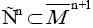an oriented connexion sub-manifold of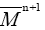and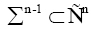a compact hyper-surface of Pn .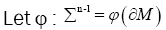a compact oriented hyper-surface of boundary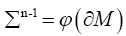Denoting by N the global vector fields normal to Mn, and ν the outpointing vector normal to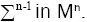Then for 1 ≤ k ≤ n and for every conformal vector field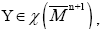we have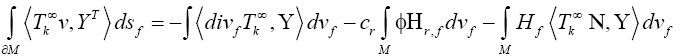Where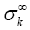is the weighted Newton transformations, and Hr,f is the weighted higher order mean curvature.

As a consequence of this proposition, we obtain some special cases. In particular, we obtain a balancing formula for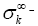minimal hyper-surface in space forms. The paper is organized as follows. Section 2 provides some preliminaries. The main results of the paper are contained in Section 3. Throughout the paper everything (manifolds, metrics, etc.) is assumed to be C -differentiable and oriented [3-5].

Preliminaries

In this section we introduce the basic notations used in the paper. we will recall some definitions and properties of the weighted symmetric functions and the weighted Newton transformations. For more details see ,.

Let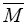be a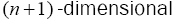Riemannian manifold. Let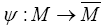be an isometrically immersed hypersurface. Denoting by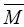andthe Levi civita connections ofandrespectively. Then, the Weingarten formulae of the hypersurface is written as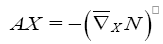Where Τ is the shape operator of the hypersurface M with respect to the Guass map N, and Τ denotes the orthogonal projection on the vector bundle tangent to M,

It is well know that A is a linear self adjoint operator. At each point p∈M, its eigenvalues μ1,..., μn are the principales curvatures of M [6,7].

Associate to the shape operator A , define the weighted elementary symmetric fucntions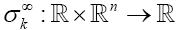recursively by: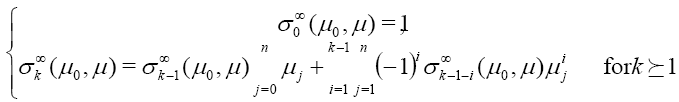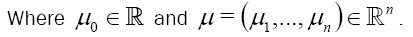In particular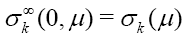is nothing but the classical elementary symmetric functions define in .

Definition

The weighted Newton transformations (W.N.T)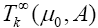are define inductively form A by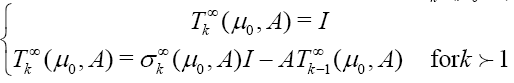Where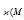denote the identity of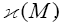Or equivalentely by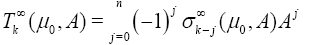Where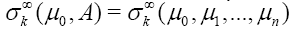and n μ ,...,μ 1 are the eigenvalues of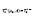We denote to simplify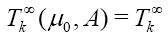and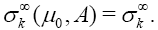In particular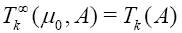is the classical Newton transformations introduced in 

These two quantities has the same proprietes of the classical symmetric functions and Newton transformations .

Proposition 2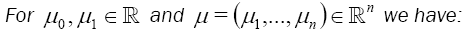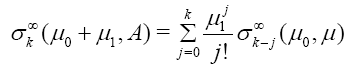In particular 1,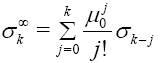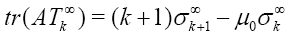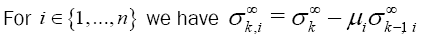And the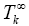eigenvalue of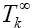is equal to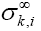Where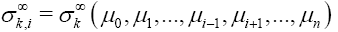Definition 1: The weighted rth mean curvature Hr,f is given by: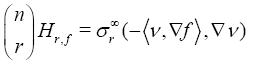Where ν is the outpointing vectors field normal to M in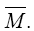In particular for r =1, we have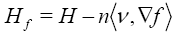Is the weighted mean curvature of the hypersurface M

Definition 2: We say that an hypersurface M of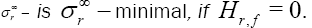In particular; M is f −minimal, if Hf= 0 . Or equivalentely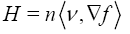Definition 3: The weighted divergence of the weighted Newton transformations is define by: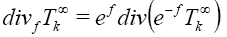Where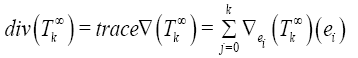And {e1,…,en} is an orthonormale basis of the tangent space of M.

In particular, if f vanish identiqualy and μ=0, then we obtain the classical difinition of the divergence of the Newton tranformations.

I found here some isoperimetrics inequalities. Now I am trying to find a variational formula for the wighted higher order mean curvature .

#### Conclusion

In conclusion, my steps are:

1. Calculate the divergence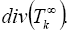(The fact that we calculate this quantity is to use it in the two last steps by using the divergence theorem for the weighte Newton transformation).

2. Compare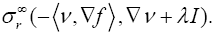3. Find variation (critical point) for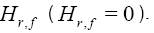4. Minimise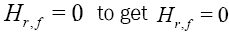Forn the two first points, I found the way.

I think the two last ones are equivalent to the study of the variation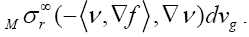If you have an idea about how we study the two last points, because if we can find such

realtion, this result gives a geometric interpretation of our quantity ( H r,f ).